Foxtable(КќБэ) Ёњ гУЛЇРИФП Ёњ зЈМвзјЬУ Ёњ ЮЪЬтЧѓжњЃЁ

ЙВга536ШЫЙизЂЙ§БОЬћЪїаЮДђгЁИДжЦСДНг

# жїЬтЃКЮЪЬтЧѓжњЃЁ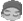lisheng1971
аЁДѓ  1ТЅ | аХЯЂ | ЫбЫї | гЪЯф | жївГ | UC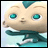МгКУгбЗЂЖЬаХ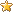ЕШМЖЃКЭЏКќ ЬћзгЃК218 Л§ЗжЃК1676 ЭўЭћЃК0 ОЋЛЊЃК0 зЂВсЃК2016/4/14 9:45:00
ЮЪЬтЧѓжњЃЁ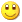Post ByЃК2018/12/7 11:17:00 [жЛПДИУзїеп]

 зђЬьЕФЬљзгВЛжЊдѕУДЛиЪТЃЌевВЛЕНСЫЃЁМЬзђЬьЮЪЬтЧѓжњЃК1ЁЂгаБэ1ЁЂБэ2ЃЌИББэAЁЂBЁЂЃЈЖМАѓЖЈЕФЪЧБэ1ЃЉЃЛ2ЁЂгавГУцКЯМЏ1ЃЌPage1жагаБэ1ЁЂPage2жагаИББэAЁЂpage3жагаИББэBЁЂpage4жагаБэ2ЃЛ3ЁЂЁАЕМШыЁБАДХЅ1ЃЌЯждкДњТыПЩЪЕЯждкPage1ЪБЕМШыЪ§ОнНјБэ1ЃЌдкPage4ЪБЕМШыЪ§ОнНјБэ2ЃЛЃЈСНЖЮДњТыГ§БэУћВЛвЛбљЃЌЦфЫќЖМвЛбљЃЁЃЉЯждкЮЪЬтЃК1ЁЂзђЬьАцжїЫЕПЩНЋДњТыЙЋЙВВПЗжаДдкЭтУцЃЌетИіЮвУЛИуУїАзЃЛ              2ЁЂдкPage2ЁЂ3ЪБЃЌШчЯыЕМШыАДХЅЦ№зїгУЃЌДњТыСПЛсКмДѓЃЌФмЗёОЋМѕЃПИНВПЗжДњТыЃКDim tb As WinForm.TabControl = e.Form.Controls("TabControl1")Dim pg As WinForm.TabPage = tb.SelectedPageIf tb.SelectedPage.Text = "МјЖЈаХЯЂЛузм" Then    Dim Result As DialogResult    Result = MessageBox.Show("ШЗЖЈвЊЕМШыТ№?", "ЬсЪО", MessageBoxButtons.YesNo, MessageBoxIcon.Question)    If Result = DialogResult.Yes Then        Dim dlg As New OpenFileDialog        dlg.Filter = "ExcelЮФМў|*.xls"        dlg.MultiSelect = True 'дЪаэбЁдёЖрИіЮФМў        If dlg.ShowDialog =DialogResult.OK Then            Dim Book As New XLS.Book(dlg.FileName)            Dim Sheet As XLS.Sheet = Book.Sheets(0)            Tables("МјЖЈЙмРэБэ").ResumeRedraw()            Tables("МјЖЈЙмРэБэ").StopRedraw()            systemready = False            Dim nms As New Dictionary(Of String, Integer)            Dim dic As new Dictionary(Of DataRow, Integer)            Dim ls As new List(Of Integer)            For c As Integer = 0 To sheet.Cols.Count - 1                Dim str As String = sheet(1,c).Text.Replace(" ", "")                If  DataTables("МјЖЈЙмРэБэ").DataCols.Contains(str) Then                    nms.Add(str, c)                End If            Next            For n As Integer = 2 To Sheet.Rows.Count -1                Dim jdpc As String = sheet(n,6).Text                Dim sfzhm As String = sheet(n,9).Text                Dim dr As DataRow = DataTables("МјЖЈЙмРэБэ").Find("МјЖЈХњДЮ = '" & jdpc & "' And ЩэЗнжЄКХТы = '" & sfzhm & "'")                If dr Is Nothing Then                    ls.add(n)                Else                    dic.Add(dr, n)                End If            Next            For Each key As DataRow In dic.Keys                For Each m As String In nms.keys                    If DataTables("МјЖЈЙмРэБэ").DataCols(m).IsBoolean Then                        If Sheet(dic(key),nms(m)).Text = "" OrElse Sheet(dic(key),nms(m)).Text = "False" OrElse Sheet(dic(key),nms(m)).Value = 0 Then                            key(m) = False                        Else                            key(m) = True                        End If                    Else If DataTables("МјЖЈЙмРэБэ").DataCols(m).Expression > "" Then                        'БэДяЪНСа                    Else If DataTables("МјЖЈЙмРэБэ").DataCols(m).IsNumeric Then                        key(m) = val(Sheet(dic(key),nms(m)).Value)                    Else                        key(m) = Sheet(dic(key),nms(m)).Value                    End If                Next            Next            For Each l As Integer In ls                Dim ndr As DataRow = DataTables("МјЖЈЙмРэБэ").AddNew                For Each m As String In nms.keys                    If DataTables("МјЖЈЙмРэБэ").DataCols(m).IsBoolean Then                        If Sheet(l,nms(m)).Text = "" OrElse Sheet(l,nms(m)).Text = "False" OrElse Sheet(l,nms(m)).Value = 0 Then                            ndr (m) = False                        Else                            ndr (m) = True                        End If                    Else If DataTables("МјЖЈЙмРэБэ").DataCols(m).Expression > "" Then                        'БэДяЪНСа                    Else If DataTables("МјЖЈЙмРэБэ").DataCols(m).IsNumeric Then                        ndr (m) = val(Sheet(l,nms(m)).Value)                    Else                        ndr (m) = Sheet(l,nms(m)).Value                    End If                Next            Next            Tables("МјЖЈЙмРэБэ").ResumeRedraw()            systemready = True        End If    End If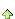lisheng1971
аЁДѓ  2ТЅ | аХЯЂ | ЫбЫї | гЪЯф | жївГ | UCМгКУгбЗЂЖЬаХЕШМЖЃКЭЏКќ ЬћзгЃК218 Л§ЗжЃК1676 ЭўЭћЃК0 ОЋЛЊЃК0 зЂВсЃК2016/4/14 9:45:00Post ByЃК2018/12/7 11:18:00 [жЛПДИУзїеп]

 етРяЃКМјЖЈаХЯЂЛузмМДPage1ЃЌМјЖЈЙмРэБэМДБэ1гаЕуЬ№
аЁДѓ  3ТЅ | аХЯЂ | ЫбЫї | гЪЯф | жївГ | UCМгКУгбЗЂЖЬаХ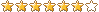ЕШМЖЃКАцжї ЬћзгЃК82764 Л§ЗжЃК414995 ЭўЭћЃК0 ОЋЛЊЃК5 зЂВсЃК2012/10/18 22:13:00Post ByЃК2018/12/7 11:43:00 [жЛПДИУзїеп]

 Dim tb As WinForm.TabControl = e.Form.Controls("TabControl1")Dim pg As WinForm.TabPage = tb.SelectedPageDim Бэ As String = ""If tb.SelectedPage.Text = "МјЖЈаХЯЂЛузм" Then    Бэ = "МјЖЈЙмРэБэ"End If   Dim Result As DialogResultResult = MessageBox.Show("ШЗЖЈвЊЕМШыТ№?", "ЬсЪО", MessageBoxButtons.YesNo, MessageBoxIcon.Question)If Result = DialogResult.Yes Then    Dim dlg As New OpenFileDialog    dlg.Filter = "ExcelЮФМў|*.xls"    dlg.MultiSelect = True 'дЪаэбЁдёЖрИіЮФМў    If dlg.ShowDialog =DialogResult.OK Then        Dim Book As New XLS.Book(dlg.FileName)        Dim Sheet As XLS.Sheet = Book.Sheets(0)        Tables(Бэ).ResumeRedraw()        Tables(Бэ).StopRedraw()        systemready = False        Dim nms As New Dictionary(Of String, Integer)        Dim dic As new Dictionary(Of DataRow, Integer)        Dim ls As new List(Of Integer)        For c As Integer = 0 To sheet.Cols.Count - 1            Dim str As String = sheet(1,c).Text.Replace(" ", "")            If  DataTables(Бэ).DataCols.Contains(str) Then                nms.Add(str, c)            End If        Next        For n As Integer = 2 To Sheet.Rows.Count -1            Dim jdpc As String = sheet(n,6).Text            Dim sfzhm As String = sheet(n,9).Text            Dim dr As DataRow = DataTables(Бэ).Find("МјЖЈХњДЮ = '" & jdpc & "' And ЩэЗнжЄКХТы = '" & sfzhm & "'")            If dr Is Nothing Then                ls.add(n)            Else                dic.Add(dr, n)            End If        Next        For Each key As DataRow In dic.Keys            For Each m As String In nms.keys                If DataTables(Бэ).DataCols(m).IsBoolean Then                    If Sheet(dic(key),nms(m)).Text = "" OrElse Sheet(dic(key),nms(m)).Text = "False" OrElse Sheet(dic(key),nms(m)).Value = 0 Then                        key(m) = False                    Else                        key(m) = True                    End If                Else If DataTables(Бэ).DataCols(m).Expression > "" Then                    'БэДяЪНСа                Else If DataTables(Бэ).DataCols(m).IsNumeric Then                    key(m) = val(Sheet(dic(key),nms(m)).Value)                Else                    key(m) = Sheet(dic(key),nms(m)).Value                End If            Next        Next        For Each l As Integer In ls            Dim ndr As DataRow = DataTables(Бэ).AddNew            For Each m As String In nms.keys                If DataTables(Бэ).DataCols(m).IsBoolean Then                    If Sheet(l,nms(m)).Text = "" OrElse Sheet(l,nms(m)).Text = "False" OrElse Sheet(l,nms(m)).Value = 0 Then                        ndr (m) = False                    Else                        ndr (m) = True                    End If                Else If DataTables(Бэ).DataCols(m).Expression > "" Then                    'БэДяЪНСа                Else If DataTables(Бэ).DataCols(m).IsNumeric Then                    ndr (m) = val(Sheet(l,nms(m)).Value)                Else                    ndr (m) = Sheet(l,nms(m)).Value                End If            Next        Next        Tables(Бэ).ResumeRedraw()        systemready = True    End IfEnd Iflisheng1971
аЁДѓ  4ТЅ | аХЯЂ | ЫбЫї | гЪЯф | жївГ | UCМгКУгбЗЂЖЬаХЕШМЖЃКЭЏКќ ЬћзгЃК218 Л§ЗжЃК1676 ЭўЭћЃК0 ОЋЛЊЃК0 зЂВсЃК2016/4/14 9:45:00Post ByЃК2018/12/7 16:29:00 [жЛПДИУзїеп]

 Dim tb As WinForm.TabControl = e.Form.Controls("TabControl1")Dim pg As WinForm.TabPage = tb.SelectedPageDim Бэ As String = ""If tb.SelectedPage.Text = "МјЖЈаХЯЂЛузм" Then    Бэ = "МјЖЈЙмРэБэ"ElseIf tb.SelectedPage.Text = "жЄЪщБрКХЛузм" Then    Бэ = "жЄЪщБрКХБэ"End If Dim Result As DialogResultResult = MessageBox.Show("ШЗЖЈвЊЕМШыТ№?", "ЬсЪО", MessageBoxButtons.YesNo, MessageBoxIcon.Question)If Result = DialogResult.Yes Then    Dim dlg As New OpenFileDialog    dlg.Filter = "ExcelЮФМў|*.xls"    dlg.MultiSelect = True 'дЪаэбЁдёЖрИіЮФМў    If dlg.ShowDialog =DialogResult.OK Then        Dim Book As New XLS.Book(dlg.FileName)        Dim Sheet As XLS.Sheet = Book.Sheets(0)        Tables(Бэ).ResumeRedraw()        Tables(Бэ).StopRedraw()        systemready = False        Dim nms As New Dictionary(Of String, Integer)        Dim dic As new Dictionary(Of DataRow, Integer)        Dim ls As new List(Of Integer)         ...................................................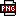ДЫжїЬтЯрЙиЭМЦЌШчЯТЃК1544171473(1).png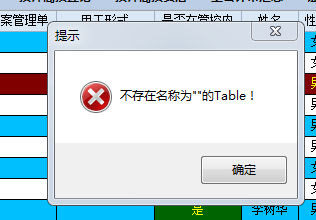lisheng1971
аЁДѓ  5ТЅ | аХЯЂ | ЫбЫї | гЪЯф | жївГ | UCМгКУгбЗЂЖЬаХЕШМЖЃКЭЏКќ ЬћзгЃК218 Л§ЗжЃК1676 ЭўЭћЃК0 ОЋЛЊЃК0 зЂВсЃК2016/4/14 9:45:00Post ByЃК2018/12/7 16:31:00 [жЛПДИУзїеп]

 ЮЊЪВУДЛсБЈДэЃПЪЧЮвДњТыаДДэСЫТ№ЃПгаЕуЬ№
аЁДѓ  6ТЅ | аХЯЂ | ЫбЫї | гЪЯф | жївГ | UCМгКУгбЗЂЖЬаХЕШМЖЃКАцжї ЬћзгЃК82764 Л§ЗжЃК414995 ЭўЭћЃК0 ОЋЛЊЃК5 зЂВсЃК2012/10/18 22:13:00Post ByЃК2018/12/7 16:41:00 [жЛПДИУзїеп]

 msgbox(tb.SelectedPage.Text) ПДЕЏГіЪВУДЃПlisheng1971
аЁДѓ  7ТЅ | аХЯЂ | ЫбЫї | гЪЯф | жївГ | UCМгКУгбЗЂЖЬаХЕШМЖЃКЭЏКќ ЬћзгЃК218 Л§ЗжЃК1676 ЭўЭћЃК0 ОЋЛЊЃК0 зЂВсЃК2016/4/14 9:45:00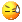Post ByЃК2018/12/7 19:06:00 [жЛПДИУзїеп]ДЫжїЬтЯрЙиЭМЦЌШчЯТЃК1544179876(1).png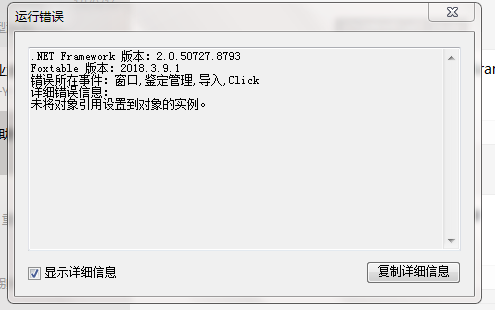ЮвевЕНОпЬхд­вђСЫЃЌЪЧЮвдквГУцЕФУћГЦЩЯДђДэСЫзжЃЌв§Ц№БЈДэЃЁАцжїЃКЯыЮЪЯТЮЊЪВУДЮвгаЪ§ОндіМѕЃЌБШШчНкЕуЪЧЁАХњДЮЁБЃЌЮвдіМгЛђМѕЩй1ХњЃЌЕЋФПТМЪїНкЕуЩЯВЛЛсЫцжЎдіМѕЃЌЖјЪЧвЊЮвЙиБеДАПкдйжиаТДђПЊВХФмПДЕНЃПlisheng1971
аЁДѓ  8ТЅ | аХЯЂ | ЫбЫї | гЪЯф | жївГ | UCМгКУгбЗЂЖЬаХЕШМЖЃКЭЏКќ ЬћзгЃК218 Л§ЗжЃК1676 ЭўЭћЃК0 ОЋЛЊЃК0 зЂВсЃК2016/4/14 9:45:00Post ByЃК2018/12/7 19:13:00 [жЛПДИУзїеп]

 ЛЙгаОЭЪЧдкФПТМЪїЩЯЮвПЊЦєЁАЯдЪОИДбЁПђЁБКѓИУдѕУДВХФмШУЫќзїгУЃЌПДАяжњКЭЪОР§ЃЌУВЫЦИњЮвЯждкЕФДњТыЭъШЋЪЧСНЛиЪТЃЌЮвЯывРКљТЋЛ­ЦАЖМУЛАьЗЈЃЛФПТМЪїПижЦЕФБэЕФ2ИіЃЌЫќУЧгаЙВЭЌЕФСаЃКЁАХњДЮЁБЁЂЁАЩэЗнжЄКХТыЁБЃЌФПТМЪїЕФНкЕувВЪЧет2ИіЁЃЧыАцжїжИЕуЃЁ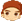гаЕуРЖ
аЁДѓ  9ТЅ | аХЯЂ | ЫбЫї | гЪЯф | жївГ | UCМгКУгбЗЂЖЬаХ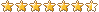ЕШМЖЃКГЌМЖАцжї ЬћзгЃК27048 Л§ЗжЃК137991 ЭўЭћЃК0 ОЋЛЊЃК7 зЂВсЃК2015/6/24 9:21:00Post ByЃК2018/12/7 20:40:00 [жЛПДИУзїеп]

 Ли7ТЅЃЌвЊжиаТЩњГЩФПТМЪїЛи8ТЅЃЌНиЭМЛђепЩЯДЋОпЬхЪЕР§ЫЕУївЊЪВУДаЇЙћlisheng1971
аЁДѓ  10ТЅ | аХЯЂ | ЫбЫї | гЪЯф | жївГ | UCМгКУгбЗЂЖЬаХЕШМЖЃКЭЏКќ ЬћзгЃК218 Л§ЗжЃК1676 ЭўЭћЃК0 ОЋЛЊЃК0 зЂВсЃК2016/4/14 9:45:00Post ByЃК2018/12/7 20:56:00 [жЛПДИУзїеп]

 ШчЭМЃКЃБЁЂШчКЮзіЕНЫцвтЙДЃБХњДЮЛђЖрХњДЮНјааВщбЏЃЈЯждкжЛФмЫЋЛїЦфжаЕФЃБИіХњДЮНјааВщбЏЃЉЃЛЁЁЁЁЁЁЃВЁЂШчКЮзіЕНЫцвтЙДЃБХњДЮЛђЖрХњДЮНјааЩОГ§ЃЈЯждкжЛФмЫЋЛїЯыЩОГ§ЕФХњДЮКѓЃЌдквГУцжаЕФБэРябЁжаШЋВПЪ§ОнНјааЩОГ§ЃЉЃЛЁЁЁЁЁЁЃГЁЂАцжїЫљЫЕЕФжиаТЩњГЩФПТМЪїЃЌУЛИуУїАзЃЛЮвАДЃВжаЕФЗНЗЈЩОГ§Ъ§ОнКѓЃЌФПТМЪїВЛЛсБфЛЏЃЌБЛЩОГ§ЕФХњДЮШдШЛФмПДЕНЁЃDim Filter As StringIf e.node.Text = "МгдиЫљгаЪ§Он" Then    Filter = ""Else    Dim dr As DataRow = e.Node.DataRow 'ЛёШЁЩњГЩДЫНкЕуЕФDataRow    Select Case e.Node.Level        Case 0            Filter ="[МјЖЈХњДЮ] = '" & dr("МјЖЈХњДЮ") & "'"        Case 1            Filter ="[МјЖЈХњДЮ] = '" & dr("МјЖЈХњДЮ") & "' And [ЙЄзїЕЅЮЛ] = '" & dr("ЙЄзїЕЅЮЛ") & "'"    End SelectEnd IfетЪЧФПТМЪїВПЗжДњТызмЪ§ 12 1 2 ЯТвЛвГ